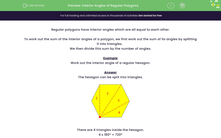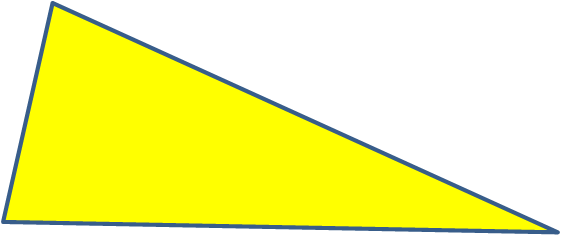# Find the Size of an Interior Angle in Regular Polygons

In this worksheet, students will calculate the interior angles of regular polygons.Key stage:  KS 3

Curriculum topic:   Geometry and Measures

Curriculum subtopic:   Derive/Use the Sum of Angles for Deduction

Popular topics:   Angles worksheets

Difficulty level:#### Worksheet Overview

You already know that the interior angles of a triangle add up to 180º.Polygons with more sides have a larger sum of interior angles.

There is a lovely little formula to use to find out the sum of the interior angle of any polygon.

Here it is:

Where n is the number of sides of a polygon: (n - 2) x 180 = the sum of the interior angles.

Let's test it out with some polygons:

Triangle has 3 sides: (3 - 2) x 180 = 1: x 180 = 180

Quadrilateral has 4 sides: (4 - 2) x 180 = 2 x 180 = 360

Pentagon has 5 sides: (5 - 2) x 180 = 3 x 180 = 540

Hexagon has 6 sides: (6 - 2) x 180 = 4 x 180 = 720

Octagon has 8 sides (8 - 2) x 180 = 6 x 180 = 1,080

Does that make sense?

This means that if we know the number of sides a shape has, we can work out the sum of the interior angles.

If we know the sum of the angles, we can also work out one individual angle if the polygon is regular, with all its angles the same size.

For example, if we know that the sum of a hexagon's angles is 720º, then all we need to do is to divide 720 by the number of angles that the hexagon has, which is 6.

720 ÷ 6 = 120º

Each of the individual interior angles in a regular hexagon is 120º.

Be careful though, this only works for a regular polygon - that is one with all its sides and angles equal in size.

Shall we move on to some questions now?

### What is EdPlace?

We're your National Curriculum aligned online education content provider helping each child succeed in English, maths and science from year 1 to GCSE. With an EdPlace account you’ll be able to track and measure progress, helping each child achieve their best. We build confidence and attainment by personalising each child’s learning at a level that suits them.

Get started